Textbook QuestionChapter 11, Problem 32P

Mott Company recently implemented a JIT manufacturing system. After one year of operation, Heidi Burrows, president of the company, wanted to compare product cost under the JIT system with product cost under the old system. Mott’s two products are weed eaters and lawn edgers. The unit prime costs under the old system are as follows:Under the old manufacturing system, the company operated three service centers and two production departments. Overhead was applied using departmental overhead rates. The direct overhead costs associated with each department for the year preceding the installation of JIT are as follows: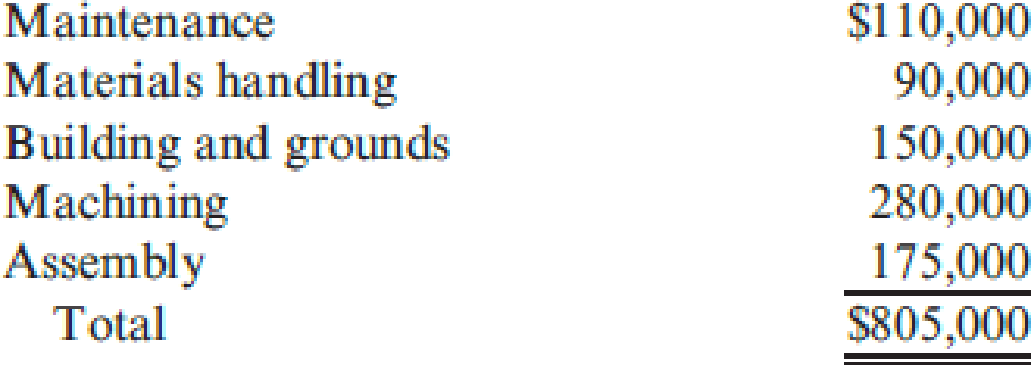Under the old system, the overhead costs of the service departments were allocated directly to the producing departments and then to the products passing through them. (Both products passed through each producing department.) The overhead rate for the Machining Department was based on machine hours, and the overhead rate for assembly was based on direct labor hours. During the last year of operations for the old system, the Machining Department used 80,000 machine hours, and the Assembly Department used 20,000 direct labor hours. Each weed eater required 1.0 machine hour in Machining and 0.25 direct labor hour in Assembly. Each lawn edger required 2.0 machine hours in Machining and 0.5 hour in Assembly. Bases for allocation of the service costs are as follows: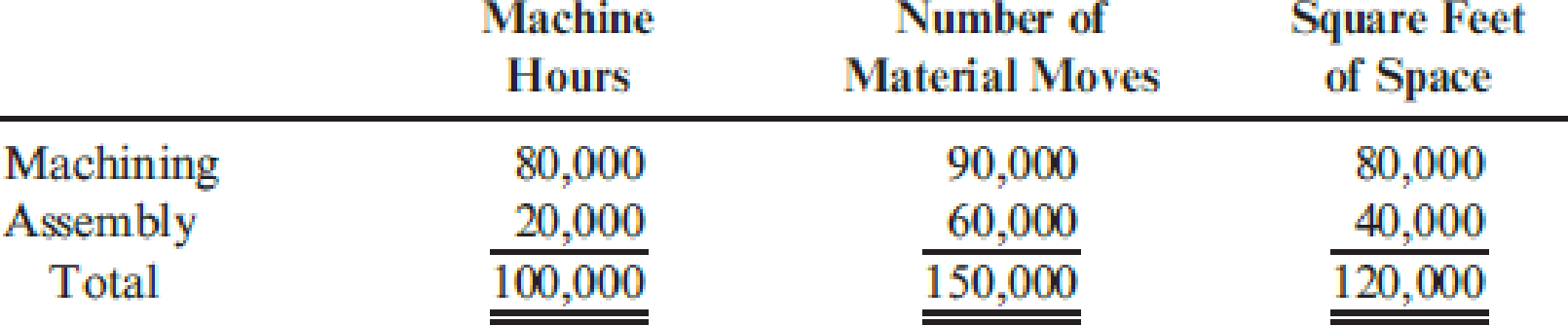Upon implementing JIT, a manufacturing cell for each product was created to replace the departmental structure. Each cell occupied 40,000 square feet. Maintenance and materials handling were both decentralized to the cell level. Essentially, cell workers were trained to operate the machines in each cell, assemble the components, maintain the machines, and move the partially completed units from one point to the next within the cell. During the first year of the JIT system, the company produced and sold 20,000 weed eaters and 30,000 lawn edgers. This output was identical to that for the last year of operations under the old system. The following costs have been assigned to the manufacturing cells: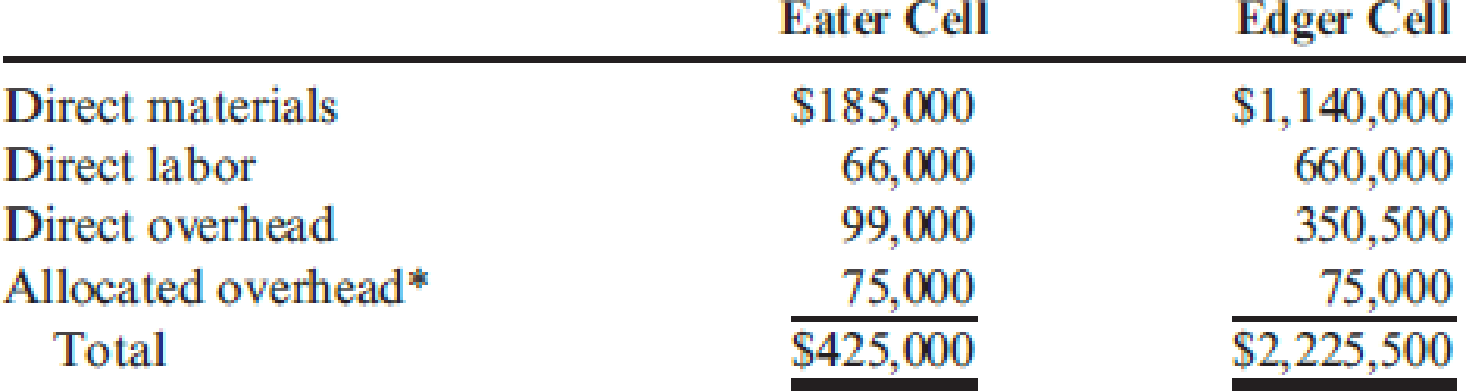Required:

1. 1. Compute the unit cost for each product under the old manufacturing system.
2. 2. Compute the unit cost for each product under the JIT system.
3. 3. Which of the unit costs is more accurate? Explain. Include in your explanation a discussion of how the computational approaches differ.
4. 4. Calculate the decrease in overhead costs under JIT, and provide some possible reasons that explain the decrease.

1.

Expert Solution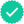To determine

Calculate the unit cost of each product under old manufacturing system.

### Explanation of Solution

Just-in-time (JIT) manufacturing system: Just-in-time (JIT) manufacturing system is an approach to manufacturing, that eliminates and reduces the non-value-added activities and it concentrates on both product quality and efficiency.

Calculate the unit cost of each product under old manufacturing system as follows:

 Particulars Eaters Edgers Direct materials \$12.00 \$45.00 Direct labor \$4.00 \$30.00 Overhead (7) \$10.07 \$20.14 Total \$26.07 \$95.14

Table (1)

Working note (1):

Calculate the allocation ratios for machining and assembly.

 Particulars Machine hour Allocation ratio Material moves Allocation ratio Square feet Allocation ratio Machining 80,000 4/5 90,000 3/5 80,000 2/3 Assembly 20,000 1/5 60,000 2/5 40,000 1/3 Total 100,000 150,000 120,000

Table (2)

Note: Formula for allocation ratio is (Individual valueTotal amount of line).

Working note (2):

Calculate the direct overhead cost of maintenance.

For machining department:

Direct overhead cost = (Total direct overhead cost of maintenance×Allocation rate in machine hours (1))=\$110,000×45=\$88,000

For assembly department:

Direct overhead cost = (Total direct overhead cost of maintenance×Allocation rate in machine hours (1))=\$110,000×15=\$22,000

Working note (3):

Calculate the direct overhead cost of material handling.

For machining department:

Direct overhead cost = (Total direct overhead cost for materials handling×Allocation rate for material moves (1))=\$90,000×35=\$54,000

For assembly department:

Direct overhead cost = (Total direct overhead cost of mateiral handling×Allocation rate in material handling (1))=\$90,000×25=\$36,000

Working note (4):

Calculate the direct overhead cost of building and grounds.

For machining department:

Direct overhead cost = (Total direct overhead cost for buildings and grounds×Allocation rate for square feet (1))=\$150,000×23=\$100,000

For assembly department:

Direct overhead cost = (Total direct overhead cost of building and grounds×Allocation rate in square feet (1))=\$150,000×13=\$50,000

Working note (5):

Calculate the total direct overhead cost for each department.

 Particulars Machining Assembly Direct overhead costs \$280,000 \$175,0000 Add: Direct overhead cost for maintenance (2) \$88,000 \$22,000 Add: Direct overhead cost for material handling (3) \$54,000 \$36,000 Add: Direct overhead cost for building and grounds (4) \$100,000 \$50,000 Total \$522,000 \$283,000

Table (3)

Working note (6):

Calculate the direct overhead cost per hour for each department.

For machining department:

Direct overhead cost per hour} = Total direct overhead costTotal machine hour=\$522,00080,000 machine hours=\$6.53 per machine hour

For assembly department:

Direct overhead cost per hour} = Total direct overhead costTotal labor hours=\$283,00020,000 labor hours=\$14.15 per labor hour

Working note (7):

Calculate the direct overhead cost per unit for each product.

For Eaters:

Direct overhead cost per unit }[(Direct overhead cost per machine hour for machining (6)×Required machine hour)+(Direct overhead cost per labor hour for assembly (6)×Required machine hour)]=(\$6.53×1)+(\$14.15×0.25)=\$10.07

For Edgers:

Direct overhead cost per unit }[(Direct overhead cost per machine hour for machining (6)×Required machine hour)+(Direct overhead cost per labor hour for assembly (6)×Required machine hour)]=(\$6.53×2)+(\$14.15×0.50)=\$20.14

2.

Expert SolutionTo determine

Calculate the unit cost of each product under JIT system.

### Explanation of Solution

Calculate the unit cost of each product under JIT system as follows:

Unit cost of Eaters under JIT:

Unit cost = Total cost of Eater cellNumber of Eaters sold =\$425,00020,000=\$21.25

Unit cost of Edgers under JIT:

Unit cost = Total cost of Edger cellNumber of Edgers sold =\$2,225,50030,000=\$74.18

3.

Expert SolutionTo determine

Identify the unit cost which is more accurate, and explain the manner in which the computational approaches differ.

### Explanation of Solution

Identify the unit cost which is more accurate, and explain the manner in which the computational approaches differ as follows:

Just in time inventory costs are more accurate because,

• The building and ground costs are assigned for activity based approach.
• All costs of company (except the building and grounds) are directly attributable to each product.

The assignment of the company should be measured at the activity based costing method, because the company traces the cost and products based on each activity of the production. Since, both cells (Eaters and Edgers) are dedicated to produce a single product, whatever causal factors that are used to allocate each cell is same as the final product.

The functional based costing system first assigns the cost to each department, and then assigns the cost to the product based on the cost drivers, but the maintenance and materials handing cost is not a unit-based activities.

4.

Expert SolutionTo determine

Ascertain the decrease in overhead costs under just in time method and given some possible reasons.

### Explanation of Solution

Ascertain the decrease in overhead costs under Just in time method and given some possible reasons as follows:

In this case, the overhead cost has been decreased by \$205,500 and the decrease in overhead cost is explained by the following factors:

• Usage of interdisciplinary labor,
• Total quality control,
• Decentralization of services and
• Physical organization of the manufacturing cell.

Working note (8):

Calculate the overhead cost under JIT.

Working note (9):

### Want to see more full solutions like this?

Subscribe now to access step-by-step solutions to millions of textbook problems written by subject matter experts!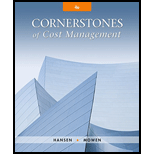Want to see more full solutions like this?Want to see more full solutions like this?
Subscribe now to access step-by-step solutions to millions of textbook problems written by subject matter experts.